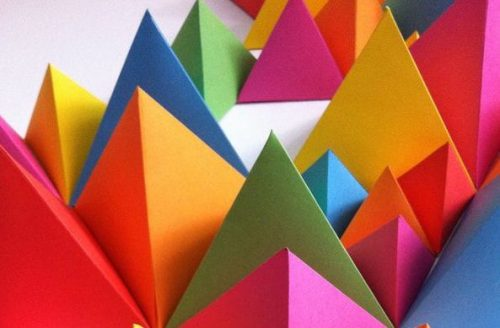# Mathematical Art Lessons

On this page you will find a selection of fully-resourced lessons with a ‘mathematical art’ theme. The activities in these lessons will help to reinforce many important mathematical skills such as accurate measuring and constructing, visual problem solving and strategic forward planning.Students can reinforce many important mathematical skills using artistic patterns and concepts. Students may find the creative activities therapeutic too!

https://www.artfulmaths.com/mathematical-art-lessons.html